• 用MATLAB求串联、并联和反馈闭环传递函数Obtaining Cascaded, Parallel, and Feedback (Closed-Loop) Transfer Functions with MATLAB . 涉及到各个传递函数系统传递函数： 一：系统是串联系统，...
用MATLAB求串联、并联和反馈闭环传递函数

Obtaining Cascaded, Parallel, and Feedback (Closed-Loop) Transfer Functions with MATLAB .

内容总结 来自于《Modern Control Engineering Fifth Edition —Katsuhiko Ogata》

涉及到的各个传递函数：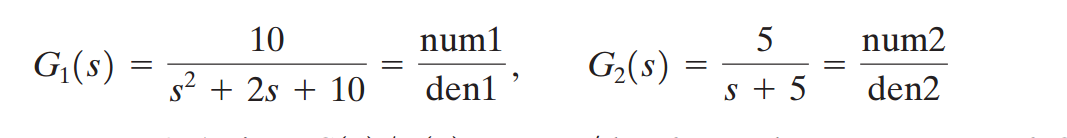系统传递函数：一：系统是串联系统，如图：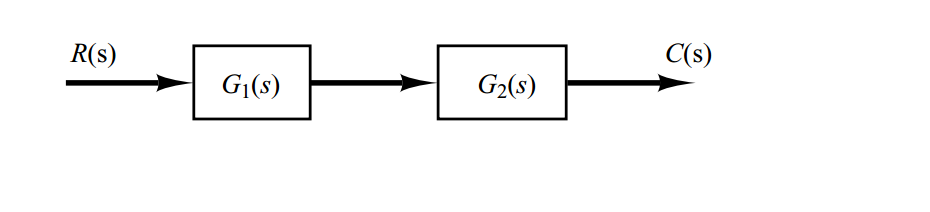利用MATLAB命令求解系统闭环传递函数：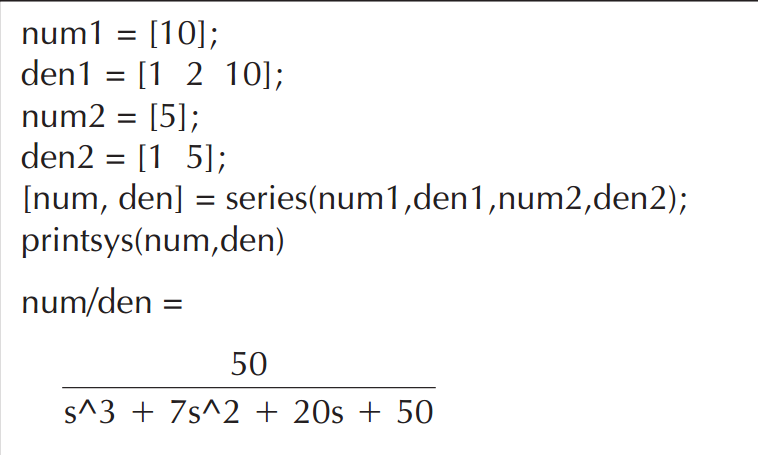二：系统是并联系统，如图：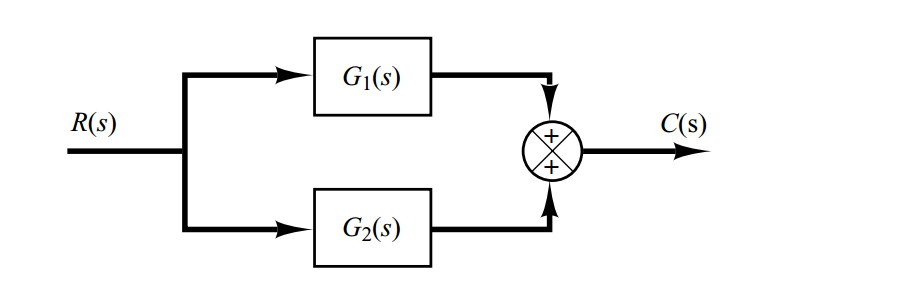利用MATLAB命令求解系统闭环传递函数：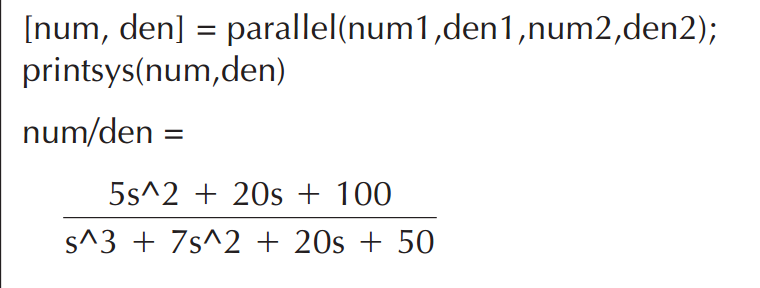二：系统是反馈系统，如图：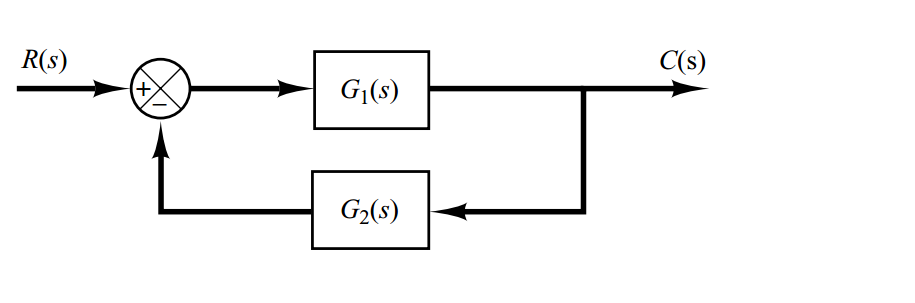利用MATLAB命令求解系统闭环传递函数：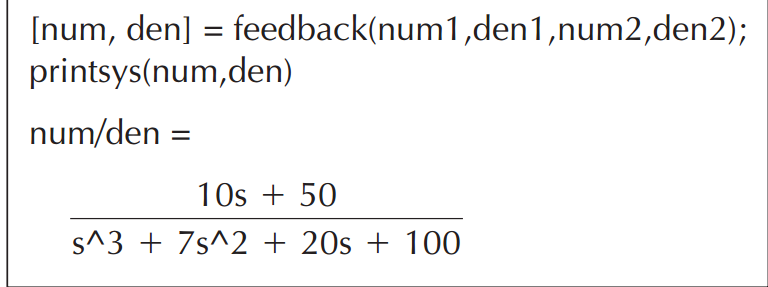展开全文Matlab现代控制
• 并联IIR结构如图1 所示，其传递函数为 ...并联系统的状态变量模型可表示为s=[A、b、c、d]，其中 　各子滤波器Hi（z）被装配成功之1阶或2阶的双二次或真接II型滤波器。 　欢迎转载，信息来自维库电
•     首先 ，我们把想要构建的传递函数分子和分母的系数按照阶次从高到低（缺项补零）分别保存在一个向量中，然后调用tf（）函数进行构建，tf函数用来建立实部或复数传递函数模型或将状态方程、或零级增益模型转化...
     本文主要介绍如何利用MATLAB编程实现系统传递函数的构建以及它们之间进行串联、并联、反馈时的构建方法
一、传递函数的构建方法
首先 ，我们把想要构建的传递函数分子和分母的系数按照阶次从高到低（缺项补零）分别保存在一个向量中，然后调用tf（）函数进行构建，tf函数用来建立实部或复数传递函数模型或将状态方程、或零级增益模型转化成传递函数形式。比如构建如下传递函数的代码如下：
$\frac{1}{s^3+2s^2+s}$
num=;
den=[1 2 1 0];
G=tf(num,den)

运行结果如下：
G =

1
---------------
s^3 + 2 s^2 + s

如果我们拿到的传递环数是以零极点的形式表示的，而我们又不想手动的去展成多项式形式，可以采用如下的方式构建传递函数，我们把传递函数的零点z，极点p，增益k，分别放到一个向量中，然后再调用zpk（）函数进行构建，如构建如下传递环数的代码如下：
$\frac{1}{s（s+1）^2}$
z=[];    //没有零点就空着，若里面写零代表分子为S
p=[0 -1 -1];
k=;
G=zpk(z,p,k)

运行结果如下：
G =

1
---------
s (s+1)^2

其实以上两种模型之间可以相互转化，转化代码如下：
   num_1=;
den_1=[1 2 1 0];
G_1=tf(num_1,den_1)
[z,p,k]=tf2zp(num_1,den_1);   //传递函数模型转化为零极点模型
G_2=zpk(z,p,k)
[num_3,den_3]=zp2tf(z,p,k);    //零极点模型转化为传递函数模型
G_3=tf(num_3,den_3)


运行结果如下：
G_1 =

1
---------------
s^3 + 2 s^2 + s

G_2 =

1
---------
s (s+1)^2

G_3 =

1
---------------
s^3 + 2 s^2 + s

二、多个传递环数间串联、并联、反馈的构建方法
本部分我们用如下两个传递函数为例，就他们之间进行串联，并联，反馈的模型搭建方法进行介绍。
$\frac{1}{s^3+2s^2+s} 和 \frac{1}{s^2+2s+1}$
1、串联
方法一：先根据第一部分的介绍搭建两个传递函数，然后利用用series函数计算两个传递函数的串联形式，代码如下：
  num_1=;
den_1=[1 2 1 0];
G_1=tf(num_1,den_1);

num_2=;
den_2=[1 2 1 ];
G_2=tf(num_2,den_2);

[num_c,den_c]=series(G_1,G_2);
G_c=tf(num_c,den_c)

这种方法呢有时会出现输出参数太多 (InputOutputModel)的错误，为了简洁性和不易错性，推荐大家采用以下的第二种方法，并联和反馈也将采用如下方法介绍：
  num_1=;
den_1=[1 2 1 0];
num_2=;
den_2=[1 2 1 ];
[num_c,den_c]=series(num_1,den_1,num_2,den_2);
G_c=tf(num_c,den_c)

运行结果如下：
G_c =

1
-------------------------------
s^5 + 4 s^4 + 6 s^3 + 4 s^2 + s

2、并联
采用parallel（）函数可以计算两个传递函数的并联形式，代码如下：
   num_1=;
den_1=[1 2 1 0];
num_2=;
den_2=[1 2 1 ];
[num_b,den_b]=parallel(num_1,den_1,num_2,den_2);
G_b=tf(num_b,den_b)

运行结果如下：
G_b =

s^3 + 3 s^2 + 3 s + 1
-------------------------------
s^5 + 4 s^4 + 6 s^3 + 4 s^2 + s

3、反馈
采用feedback（）函数可以计算两个传递函数的反馈形式，相比于前两种情况，feedback（）多了最后一个参数，为1时为正反馈，为-1时为负反馈，缺省时为负反馈，代码如下：
   num_1=;
den_1=[1 2 1 0];
num_2=;
den_2=[1 2 1 ];
[num_f,den_f]=feedback(num_1,den_1,num_2,den_2,-1); //此处为负反馈，将-1改为1，则变成正反馈
G_f=tf(num_f,den_f)

运行结果如下：
G_f =

s^2 + 2 s + 1
-----------------------------------
s^5 + 4 s^4 + 6 s^3 + 4 s^2 + s + 1

用以上方法就可以得到系统的闭环传递环数，也就得到了系统的闭环特征方程，可以进步求解特征方程的特征根，从而判断系统的稳定性，具体步骤可参考如下博文：博文链接：利用MATLAB解特征方程，并画出特征根的分布，便于分析系统的稳定性。


展开全文matlab c语言 人工智能
• 建立VOS控制器二阶系统传递函数并联同步等效模型，分析了其稳定性与稳定条件。负载阻抗变化会影响并联逆变器输出稳定，设计EXS模块基于功率均分状态补偿负载电流扰动，提高了输出电压波形质量。仿真结果验证了...
• 自动控制原理-信号流图与系统状态变量传递函数之间联系如此紧密实例分析：带有正反馈，负反馈，信号线交叉结构图简化：比较点ab之间是一个并联方框连接，引出点1夹在两个比较点a,b之间，G1G2无法并联简化，需要把...
自动控制原理-信号流图与系统状态变量传递函数之间联系如此紧密实例分析：带有正反馈，负反馈，信号线交叉的结构图简化：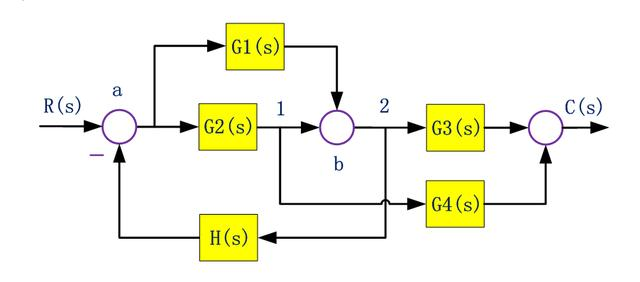比较点ab之间是一个并联方框连接，引出点1夹在两个比较点a,b之间，G1G2无法并联简化，需要把引出点1移动。引出点不能与比较点交换，因此只能往前移动到a后面、G2前面的位置；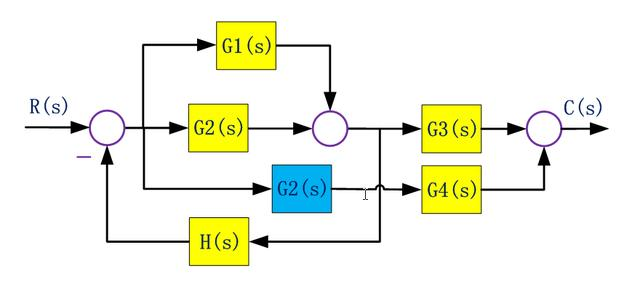把G1,G2并联方框简化，把G2G4串联方框简化，没有标明符号的比较点都是默认正反馈：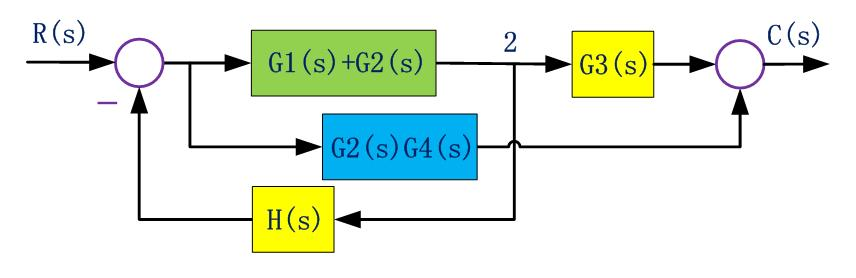把引出点2前移，使得H负反馈回路不交叉：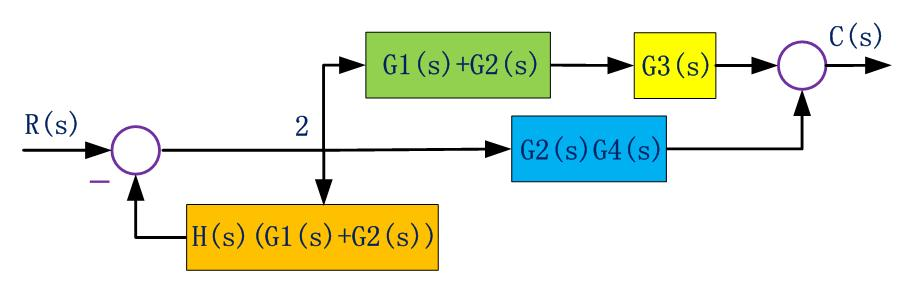简化负反馈连接方框：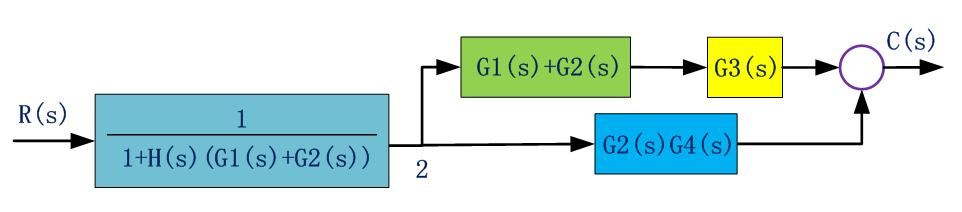由此，简化后方框之间的连接关系是简单的串并联关系，最后化简为：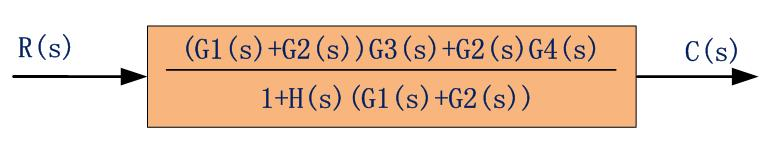信号流图的组成及其性质信号流图起源于梅森利用图示法来描述一个或一组线性代数方程式，他是由节点和支路组成的一种信号传递网络；节点用小圆圈表示，代表方程式中的变量。支路是连接两个节点的定向线段，用支路增益表示方程式中两个变量的因果关系，因此把支路称为乘法器。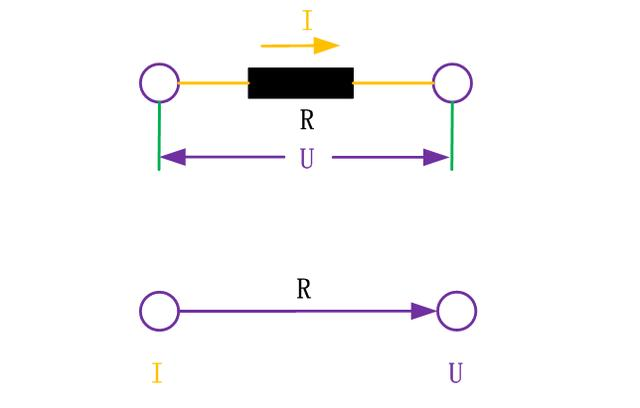以电阻元件为例，电阻流过的电流为I，电阻两端的电压为U，电阻为R。电阻满足欧姆定律：U=IR。如果定义电流I为输入，输出为电压U，支路增益为R，就可以用信号流图形式表示电阻元件；归纳：1 节点标志系统的变量。每个节点标志的变量是所有流向该节点的信号之代数和。从同一节点流向各支路的信号均用该节点的变量表示。2 支路相当于乘法器，信号流经支路时，被乘以支路增益而变换为另一信号。3 信号在支路上只能沿箭头方向传递。4 对于给定的系统，节点变量的设置是任意的，信号流图不唯一。术语：源节点(输入节点)：在源节点上，只有信号输出的支路，而没有信号输入的支路，它一般代表系统的输入变量，故也称为输入节点。阱节点(输出节点)：在阱节点上，只有输入支路， 没有输出支路，一般代表输出变量故也称为输出节点；混合节点：既有输入支路又有输出支路。前向通道：信号从输入节点到输出节点传递时，每个节点只通过一次的通路。前向通路上各支路增益乘积称为前向通道总增益。回路：起点和终点在同一个节点，而且信号通过每一个节点不多于一次的闭合通路。不接触回路：回路之间没有公共节点。信号流图绘制：任何线性数学方程都可以用信号流图表示，对于含有微分或积分的线性方程，通常经过拉氏变换，允许考虑非零初始条件，将微分方程变换为s的代数方程后再画信号流图。绘图步骤：对系统中每个变量指定一个节点，按变量因果关系从左到右顺序排列；用标明支路增益的支路，根据数学方程式将各节点变量正确连接。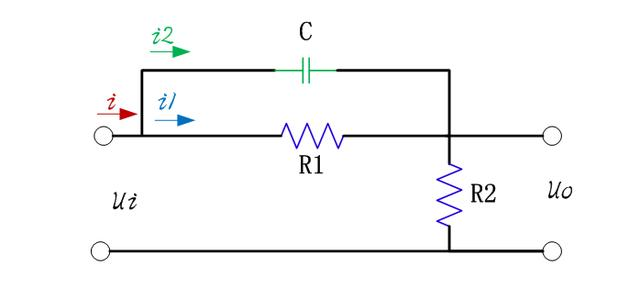已知系统结构图：信号线上标记传递的信号，方框是对变量进行变换或运算的算子。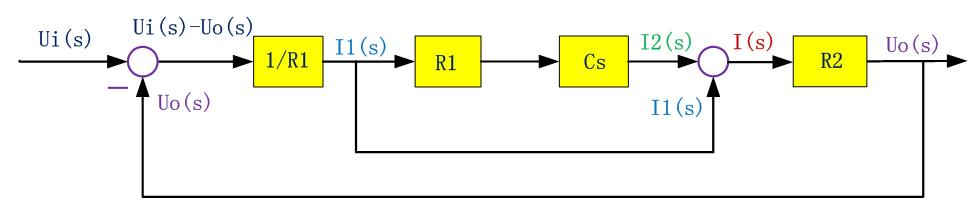设电容C两端电压为u1(t),考虑初始电压u1(0),等式求取拉氏变换，可求出非初始条件下，电容电流I2(s)：绘制信号流图：把结构图中信号线用小圆圈代替，表示传递的信号，方框用标有传递函数的有向线段代替，表示带有增益的支路。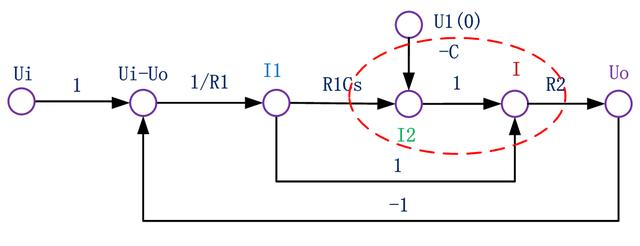注意，在比较点之前有比较点或引出点的情况，需要各设置一个节点，分别标志两个变量，它们之间的支路增益是1。梅森增益公式：利用梅森Mason增益公式直接求取从源节点到阱节点的传递函数。当不考虑非零初始条件时，也可用Mason公式计算系统结构图的传递函数。梅森增益公式可以用克莱姆法则求解线性方程组获得；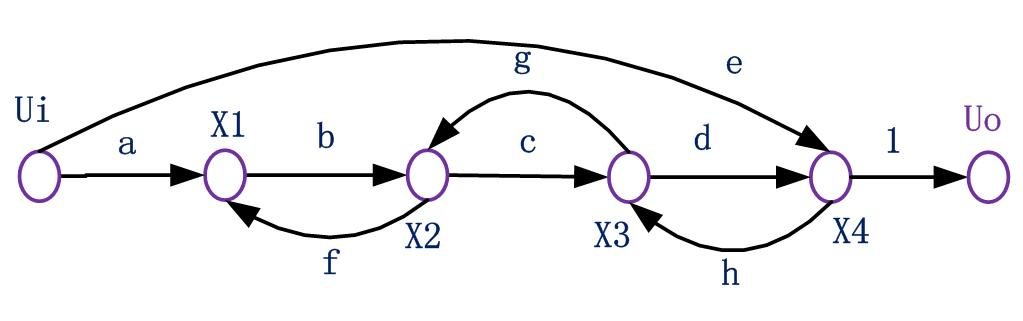根据信号流图，列写变量代数方程：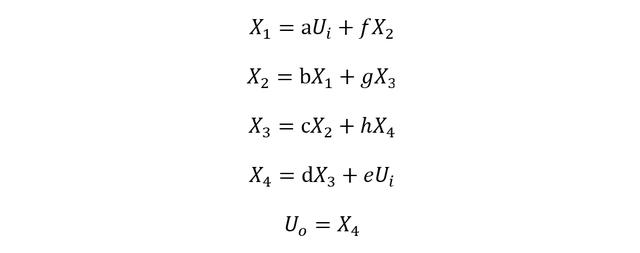把输入量提到公式右侧，左侧为待求变量：对于线性系统，可直接求取上述非齐次线性方程的解：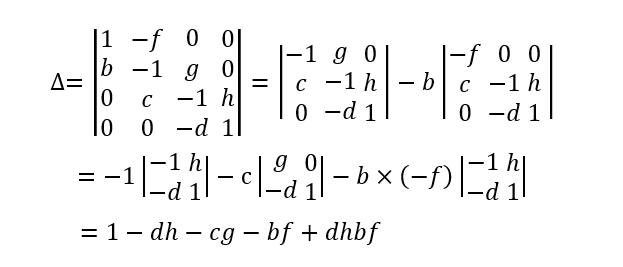方程系数矩阵行列式值：用方程右侧自由项替换矩阵第四列，求对应行列式值：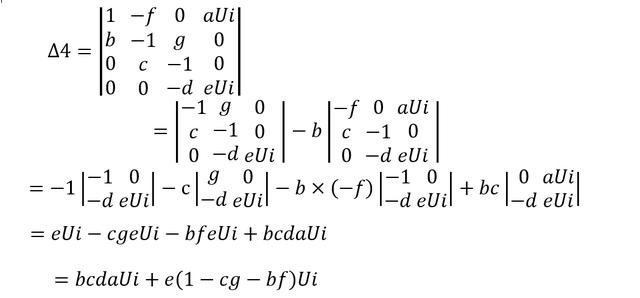由克莱姆法则可求出X4及系统传递函数：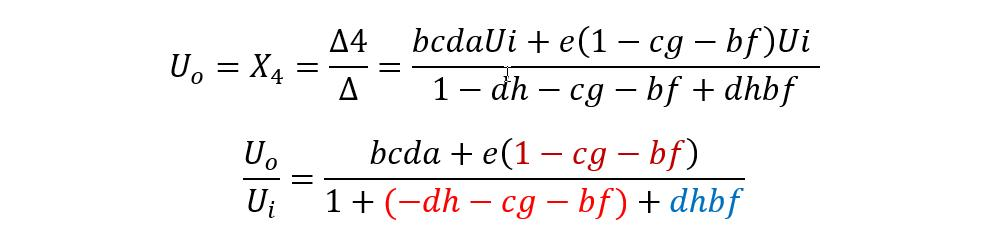梅森公式具有一般性：1 传递函数分母多项式是系统参数构成的系数行列式，分子多项式用自由项替换与输出变量对应的列向量对应的行列式。2 分母多项式包含所有独立回路增益之和，所有互不接触单独回路增益乘积之和，增益符号为-1，N个单独回路增益相乘相当于符号为(-1)N。比如上式中，分母多项式的dh,cg,bf前的符号为负，dhbf前的符号为正。3 分子多项式包含前向通道总增益减去与前向通道不接触回路的回路增益乘以该前向通道总增益，简化表达式为前向通道总增益乘以分母多项式去掉所有与该前向通道接触的回路增益项后的余项式；比如上式中，分子多项式所包含的前向通道增益e，与cg和bf不接触，因此可以把分母多项式中包含的dh看做为0，去掉与0相关的所有项后，获得不接触回路余项式为1+(-cg-bf)用梅森公式计算系统传递函数：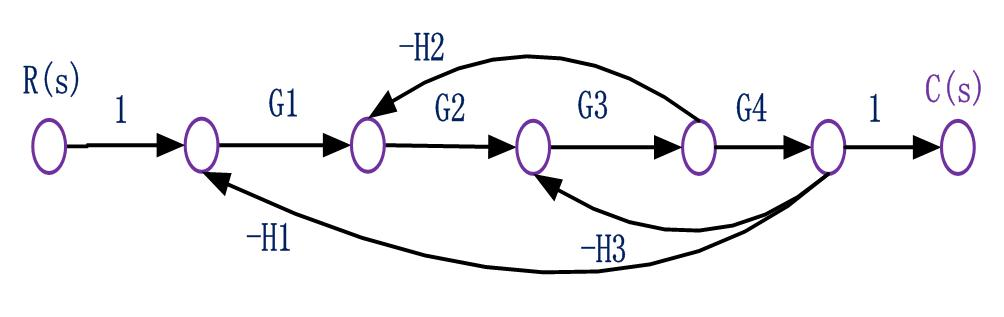这个系统有一个前向通道，三个接触单独反馈回路；因此，分子多项式只包含前向通道增益，余因子式为1；分母多项式：所有回路互相接触，因此只包含所有单独回路的增益之和；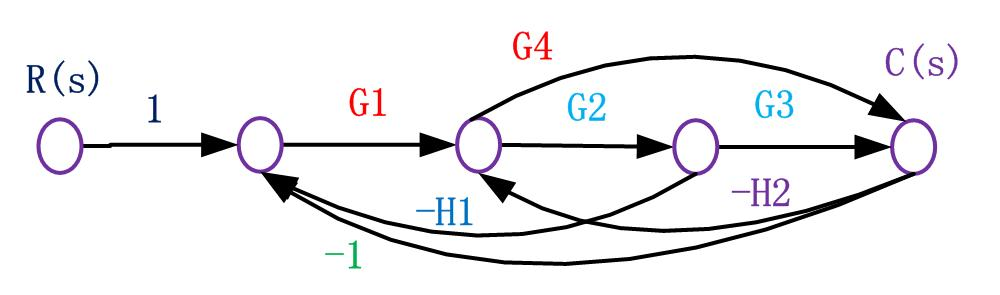这个信号图含有两个前向通道，前向通道增益分别为P1=G1G2G3,P2=G1G4;有五个单独回路，与前向通道G1G2G3相关的独立回路L1=-G1G2H1,L2=-G2G3H2,L3=-G1G2G3,与前向通道G1G4相关的独立回路：L4=-G4H2,L5=-G1G4；不接触回路为零；分子多项式为：两个前向通道的余因子式都为1分母多项式为：所有回路互相接触，因此只包含所有单独回路的增益之和；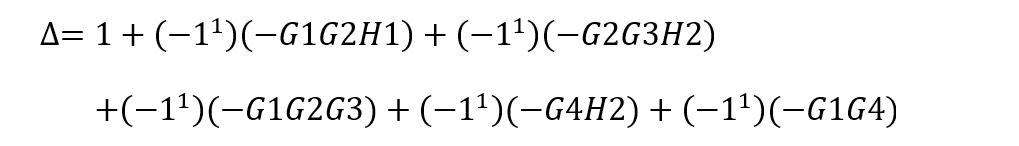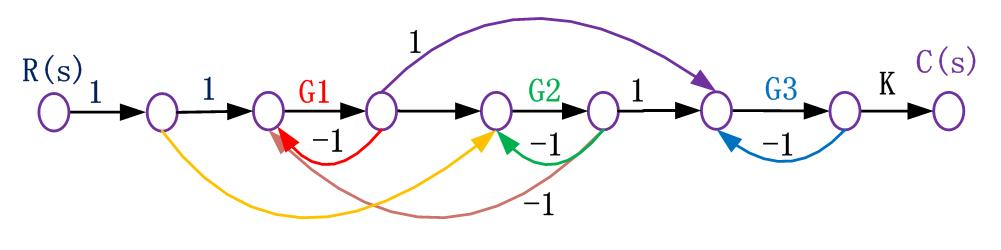这个信号图中，单独回路有四个：G1，G2，G3，G1G2两两互不接触回路有四组：三个互不接触回路有一组：因此，分母多项式为：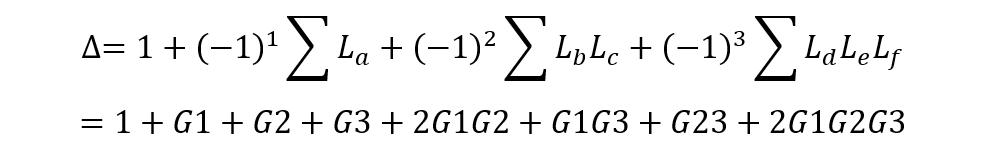从源节点R到阱节点C的前向通道共有四条，前向通道增益及余因子式分别为：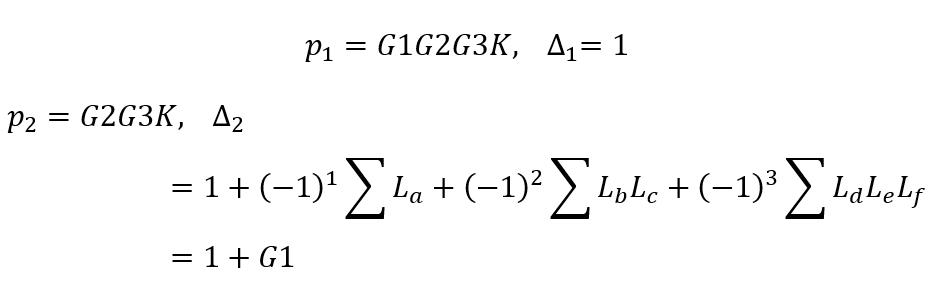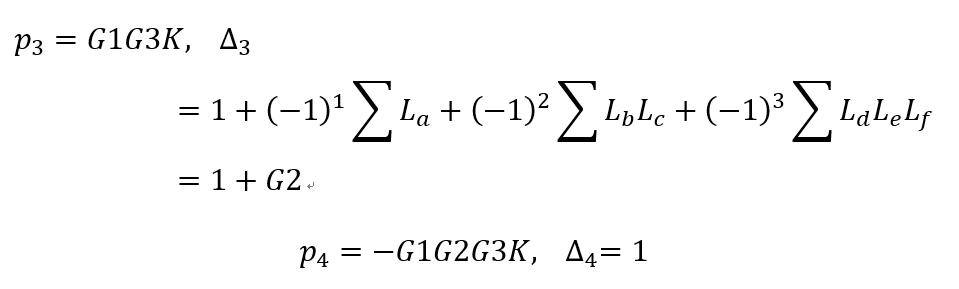第四个前向通道比较特殊，不接触回路为零。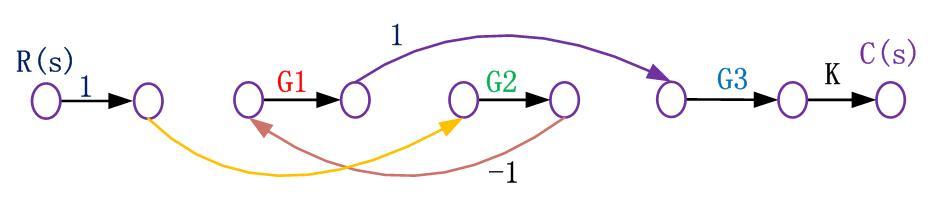系统传递函数为：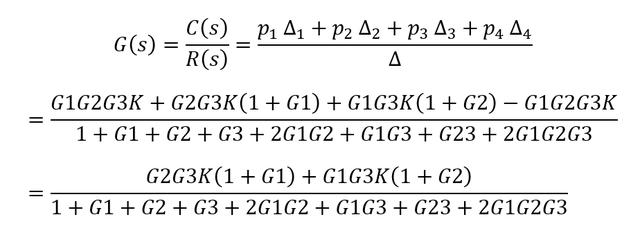用梅森公式校验系统结构图的传递函数：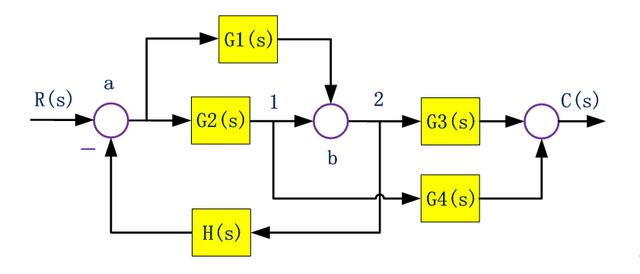把系统结构图转换为信号流图：在系统结构图的信号线上，用小圆圈标注各变量对应的节点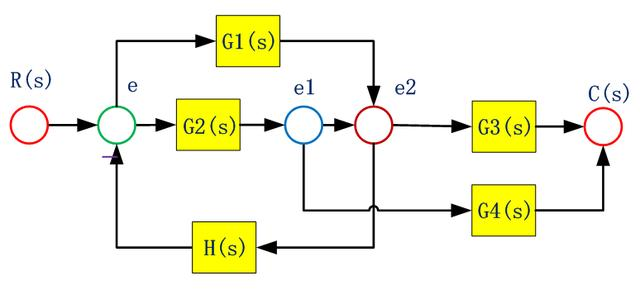将各节点按原来顺序自左向右排列，把方框用带有增益的支路代替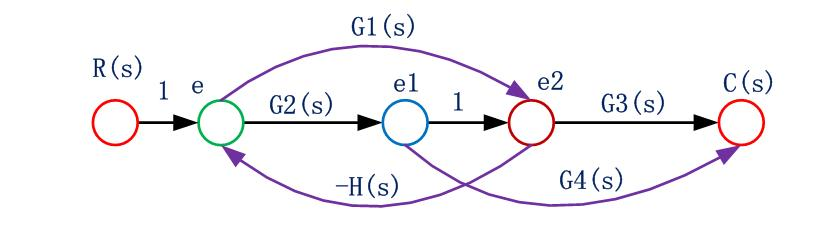系统存在2个单独回路G2H,G1H，3个前向通道G1G3,G2G3,G2G4。不接触回路为零。从源节点R到阱节点C的前向通道共有3条，前向通道总增益及余因子式分别为：单独回路有2个：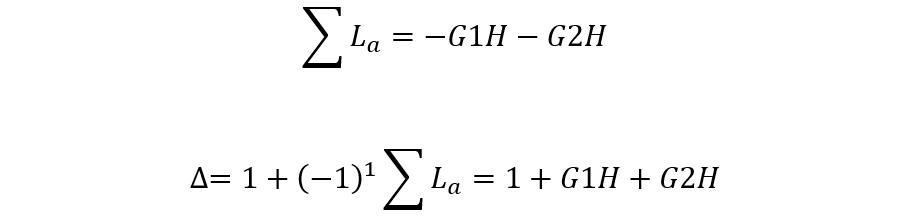获得系统传递函数：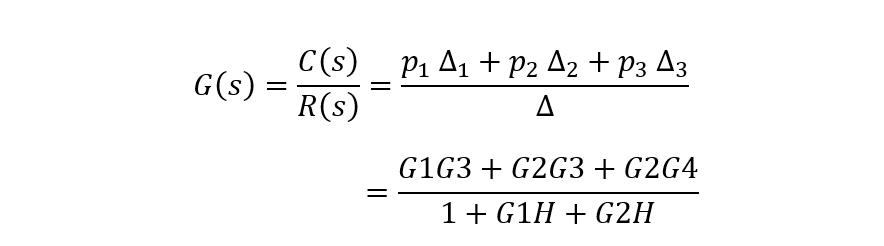可见，运用梅森公式可以非常方便的计算系统传递函数，省去了结构图的简化过程。
展开全文两个变量相乘
• 针对风电场汇流站并联静止无功发生器(SVG)可能发生机间谐波环流问题，以级联型H桥SVG并联系统为研究对象，依托系统等效电路分析机间谐波环流发生机理，并推导谐波环流解析式。基于单极倍频载波移相调制原理分析...
• 常用的simulink控制工具箱的函数 ...系统的瞬态性能指标 1.构建基本的模型函数 1.模型的表示 ​ 模型的表示有3种基本形式： 传递函数分子/分母多项式模型 传递函数零极点增益模型 状态空间模型 (1)传递函数
常用的simulink控制工具箱的函数1.构建基本的模型函数1.模型的表示(1)传递函数分子/分母多项式模型(2)传递函数零极点增益模型(3)状态空间模型：2.系统建模(1)串联(2)并联(3)反馈2.时域响应1.脉冲响应：2.阶跃响应：3.一般响应：4.系统的瞬态性能指标3.频率特性的分析(1)画$Nyquist$图(2)$Bode$图的绘制(3)频率特征量4.系统稳定性分析(1)系统特征根(2)系统的相对稳定性5.系统校正(1)相位超前校正(2)相位滞后校正(3)相位滞后超前校正(4)$PID$校正(5)$Matlab$代码6.相轨迹图的绘制(1)四阶$Runge-Kutta$法(2) 相轨迹图的绘制7.线性离散系统(1)$Z$变换的定义(2)$Z$变换的性质1.线性性质：2.延迟定理：3.超前定理：4.初值定理：5.终值定理：(3)$Z$变换表(4)$Matlab$函数(5)$Matlab$实现代码
1.构建基本的模型函数
1.模型的表示
​	模型的表示有3种基本形式：

传递函数分子/分母多项式模型
传递函数零极点增益模型
状态空间模型

(1)传递函数分子/分母多项式模型
$G(s)=\frac{b_ms^m+b_{m-1}s^{m-1}+...+b_1s+b_0}{a_ns^n+a_{n-1}s^{n-1}+...a_1s+a_0}$
matlab代码：
$num = [b_m,b_{m-1},...b_1,b_0],den = [a_n,a_{n-1},...a_1,a_0]\\ G = tf(num,den);$
(2)传递函数零极点增益模型
$G(s) = K\frac{(s-z_0)(s-z_1)...(s-z_m)}{(s-p_0)(s-p_1)...(s-p_n)}$
matlab代码：
$z = [z_0 ,z_1,...z_m]\\ p = [p_0,p_1,...p_n]\\ k = [K]\\ G = zpk(z,p,k)$
复杂函数用$conv(x1,x2)$实现连个向量卷积，用来求多项式乘法。
(3)状态空间模型：
$\dot{X}=AX+Bu\\ Y=CX+Du$
matlab代码：
$ss(A,B,C,D)$
2.系统建模
(1)串联$T(s)=\frac{Y(s)}{R(s)}=\frac{num}{den}=G_1(s)G_2(s)\\ G_1(s) = \frac{num1}{den1}\\ G_2(s) = \frac{num2}{den2}\\ [num,den] = series(num1,den1,num2,den2);$
(2)并联$G_1(s) = \frac{num1}{den1}\\ G_2(s) = \frac{num2}{den2}\\ T(s) = \frac{Y(s)}{R(s)} = \frac{num}{den}\\ [num,den] = parallel(num1,den1,num2,den2)$
(3)反馈$sign:+1:正反馈，-1:负反馈\\ G(s)=\frac{num1}{den1}\\ H(s) = \frac{num2}{den2}\\ T(s) = \frac{Y(s)}{R(s)} = \frac{num}{den}\\ [num1,den1] = feedback(num1,den1,num2,den2,sign)\\$
状态空间模型与传递函数的转化：$[den,num]=ss2tf(F,C,G,D)$。将有理多项式转换成空间状态模型：$[F,C,G,D]=tf2ss(num,den);$
2.时域响应
1.脉冲响应：
$y$:输出响应，$T$:仿真时间，$x$:状态响应（状态空间模型），$sys$:模型，$t$:仿真时间
$[y,T,x] = impulse(sys,t);$
2.阶跃响应：
$y$:输出响应，$T$:仿真时间，$x$:状态响应（状态空间模型），$sys$:模型，$t$:仿真时间
$[y,T,x] = step(sys,t);$
3.一般响应：
$y$:输出响应，$T$:仿真时间，$x$:状态响应（状态空间模型），$sys$:模型，$t$:仿真时间,$u$:输入
$[y,T,x]= lsim(sys,u,t);$
4.系统的瞬态性能指标
求$G(s) = \frac{50}{0.05s^2+(1+50\tau)s+50}$在不同的$\tau$时的单位阶跃响应：
上升时间函数:
function  trl  = trlf( x,yss,t0 )
%trl 计算上身时间
%   此处显示详细说明
r = 1;
while x(r)<yss
r = r+1;
end
trl  = (r-1)*t0;
end

峰值时间函数：
function tpl = tplf( x,t0 )
%tpl 计算峰值时间
%   此处显示详细说明
[ymax,tp] = max(x);
tpl = (tp-1)*t0;
end

最大超调量和调整时间函数：
function [tsl,mpl] = mplf( x,t0,yss ,dlta)
%mplf 计算1001个采样点时的调整时间和最大超调量
%   此处显示详细说明
[ymax,tp] = max(x);
mpl = (ymax-yss)/yss;
s = 1001;
while (x(s)>(1-dlta))&&(x(s)<1+dlta)
s = s-1;
end
tsl = (s-1)*t0;
end


主函数：
t = 0:0.001:1;
yss = 1;
dta = 0.02;
nG = [50,50,50];
tao = [0,0.0125,0.025];
trl = zeros(3,1);%上升时间
tpl = zeros(3,1);%峰值时间
mpl = zeros(3,1);%最大超调量
tsl = zeros(3,1);%调整时间
for i = 1:3
dG(i,:) = [0.05,1+50*tao(i),50];
G(i) = tf(nG(i),dG(i,:));
end
out1 = step(G(1),t);
out2 = step(G(2),t);
out3 = step(G(3),t);
%以下是计算上升时间
trl(1)  = trlf( out1,yss,0.001 );
trl(2)  = trlf( out2,yss,0.001 );
trl(3)  = trlf( out3,yss,0.001 );
%以下是计算峰值时间
tpl(1) = tplf( out1,0.001 );
tpl(2) = tplf( out2,0.001 );
tpl(3) = tplf( out3,0.001 );
%以下是计算最大超调量和调整时间
[tsl(1),mpl(1)] = mplf(out1,0.001,yss,dta);
[tsl(2),mpl(2)] = mplf(out2,0.001,yss,dta);
[tsl(3),mpl(3)] = mplf(out3,0.001,yss,dta);
%输出
disp('tao = 0 时的上身时间 峰值时间 最大超调量 调整时间： ');
disp([trl(1),tpl(1),mpl(1),tsl(1)]);
disp('tao = 0.0125 时的上身时间 峰值时间 最大超调量 调整时间： ');
disp([trl(2),tpl(2),mpl(2),tsl(2)]);
disp('tao = 0.025 时的上身时间 峰值时间 最大超调量 调整时间： ');
disp([trl(3),tpl(3),mpl(3),tsl(3)]);

结果：
>> abc
tao = 0 时的上身时间 峰值时间 最大超调量 调整时间：
0.0640    0.1050    0.3509    0.3530

tao = 0.0125 时的上身时间 峰值时间 最大超调量 调整时间：
0.0780    0.1160    0.1523    0.2500

tao = 0.025 时的上身时间 峰值时间 最大超调量 调整时间：
0.1070    0.1410    0.0415    0.1880


3.频率特性的分析
(1)画$Nyquist$图
$re:$时频特性，$im:$虚频特性，$w:$频率范围，$sys:$模型，$w$可选频率
$[re,im,w]=nyquist(sys,w);$
例如：绘制$G(s)=\frac{24(0.25s+0.5)}{(5s+2)(0.05s+2)}$的$Nyqusit$图：
k = 24;
numG = k*[0.25 0.5];
denG = conv([5,2],[0.05,2]);
sys = tf(numG,denG);
[m,n] = size(re);
[re,im] = nyquist(sys);
for i = 1:n
re1(i) = re(1,1,i);
im1(i) = im(1,1,i);
end
plot(re1,im1);grid on;
xlabel('实部');
ylabel('虚部');
title('系统Nyquist图');(2)$Bode$图的绘制
$mag:$幅频特性，$phase:$相频特性，$w:$频率范围，$sys:$模型
$[mag,phase,w]=bode(sys,w);$
例如：绘制$G(s)=\frac{24(0.25s+0.5)}{(5s+2)(0.05s+2)}$的$Bode$图：
k = 24;
numG = k*[0.25 0.5];
denG = conv([5,2],[0.05,2]);
sys = tf(numG,denG);
[m,n] = size(re);
w = logspace(-2,3,100);
bode(sys,w);
title('系统Nyquist图');(3)频率特征量
k = 24;
numG = k*[0.25 0.5];
denG = conv([5,2],[0.05,2]);
sys = tf(numG,denG);
w = logspace(-2,4,100);
[Gm,Pm,w]=bode(sys,w);
[m,n] = size(Gm);
for i = 1:n
Gm(i) = Gm(1,1,i);
Pm(i) = Pm(1,1,i);
end
[Mr,k] = max(Gm);
disp('协震峰值是：');
disp(20*log10(Mr));
disp('谐振频率是：');
disp(w(k));
n = 1;
while 20*log10(Gm(n))>=-3
n = n+1;
end
disp('截至频率是：');
disp(w(n));

结果：
协震峰值是：
9.5398

谐振频率是：
0.0100

截至频率是：
3.5112

4.系统稳定性分析
(1)系统特征根
已知特征方程$a_ns^n+a_{n-1}s^{n-1}+...+a_1s+a_0$的特征系数是$[a_n,a_{n-1},...a_1,a_0]$，求根的方法：
$roots([a_n,a_{n-1},...a_1,a_0]);$
(2)系统的相对稳定性
$sys:$系统模型，$Gm:$幅值裕度，$P_m:$相位裕度，$W_{cg}:$相位穿越频率，$W_{cp}:$幅值穿越频率。
$[G_m,P_m,W_{cg},W_{cp}]=margin(sys);\\ [mag,phase,w]=bode(sys);\rightarrow[G_m,P_m,W_{cg},W_{cp}]=margin(mag,phase,w)$
5.系统校正
(1)相位超前校正$T=R_1C_1\\\alpha = \frac{R_1}{R_1+R_2}<1\\ G(s) = \frac{U_0(s)}{U_i(s)}=\frac{1+Ts}{1+\alpha Ts}\\$
(2)相位滞后校正$\beta=\frac{R_3+R_4}{R_4}>1\\ T = R_4C_2\\ G(s) = \frac{1+Ts}{1+\beta Ts}$
(3)相位滞后超前校正$T_1 = R_1C_1,T_2 = R_2C_4\\ \beta=\frac{R_1+R_2}{R_2}>1\\ G(s) = \frac{(T_1s+1)(T_2s+1)}{(\frac{T_1}{\beta}s+1)(\beta T_2s+1)}$
(4)$PID$校正$T_i=R_1C_1+R_2C_2\\ T_d = \frac{R_1C_1R_2C_2}{R_1C_1+R_2C_2}\\ K_p=\frac{R_1C_1+R_2C_2}{R_1C_2}\\ G(s)=K_p(1+\frac{1}{T_is}+T_ds)$
(5)$Matlab$代码
function y = PID_fun(num,den,kp,ki,kd,ts,numbers,yd,M)
%PID_fun 阶跃信号
%  num:连续函数分子
%  den:连续函数分母
%  k_p,k_i,k_d:PID参数
%  ts:采样时间
%  numbers:采样点数目
%  yd:输入信号
%  M:阈值
% clc;
% clear;
sys = tf(num,den);
dsys = c2d(sys,ts,'z');
[numz,denz] = tfdata(dsys,'v');
u_1 = 0;u_2 = 0;u_3 = 0;
y_1 = 0; y_2 = 0; y_3 = 0;
x = [0 0 0]';
error_1 = 0;
error_2 = 0;
for k = 1:1:numbers
time(k) = k*ts;
du(k) = kp*x(1)+kd*x(2)+ki*x(3);
u(k) = u_1 + du(k);
if u(k)>=M
u(k) = M;
end
if u(k)<=-M
u(k) = -M;
end
y(k) = (-denz(2)*y_1 - denz(3)*y_2+numz(2)*u_1+numz(3)*u_2)/denz(1);%z变换延迟定理
error = yd(k) - y(k);
u_3 = u_2;u_2 = u_1;u_1 = u(k);
y_3 = y_2;y_2 = y_1;y_1 = y(k);
x(1) = error - error_1;
x(2) = error - 2*error_1+error_2;
x(3) = error;

error_2 = error_1;
error_1 = error;
end
figure;
plot(time,yd,'r',time,y,'b','linewidth',2);
xlabel('时间(s)');ylabel('y_d,y');
grid on
title('增量式PID响应曲线');
legend('理想位置信号','位置追踪');
end

调用
>> y = PID_fun([40 10],[1 50 0],8,0.1,10,0.001,1000,2*ones(1000,1),10);反馈校正和顺馈校正此处就不再赘述了。

6.相轨迹图的绘制
(1)四阶$Runge-Kutta$法
​	设$t$为自变量时间，$y$为因变量，微分方程的形式$\dot{y}=f(t,y)$。$'odefun'$:包含微分方程(组)的$M$文件，$ts:$自变量取值，$y_0:$变量$y$的初值，$p_{i}:$文件内的附加参数。
$[t,y] = ode45('odefun',t_s,y_0,[],p_1,p_2,...);$
(2) 相轨迹图的绘制
​	做出$\ddot{x}+0.5\dot{x}+2x+x^2=0$在初值为$(-2.2,0.5)^T$时的相轨迹图：
​	令$x_1 = x,x_2=\dot{x_1}$:
$\dot{x_1} =x_2\\ \dot{x_2} = -0.5x_2-2x_1-x_1^2$
函数：
function y = odefun1( t,x,p1 )
%UNTITLED 此处显示有关此函数的摘要
%   此处显示详细说明
y = [x(2);-p1*x(2)-2*x(1)-x(1)^2];
end

主函数：
t = 0:0.01:100;
x0 = [-2.2;0.5];
p1 = 0.5;
[t,y] = ode45(@odefun1,t,x0,[],p1);
plot(y(:,1),y(:,2),'linewidth',2);
grid on;xlabel('x_1');ylabel('x_2');
title('相轨迹图');7.线性离散系统
(1)$Z$变换的定义
​	连续信号$x(t)$,采样输出信号$x^*(t)$,单位脉冲序列$\delta_s(t)$,采样周期$T$
$x^*(t)=\sum_{n=0}^{\infty}x(nT)\delta(t-nT)\\ 当n \geq 0时，对上面进行Laplace变换：\\ L[x^*(t)]=\sum_{n=0}^{\infty}x(nT)\int_0^{\infty}\delta(t-nT)e^{-st}dt=\sum_{n=0}^{\infty}x(nT)e^{-snT}$
如果我们让$z=e^{sT}$得到$Z$变换的变换式：
$Z[x(t)]=X(z)=\sum_{n=0}^{\infty}x(nT)z^{-n}$
(2)$Z$变换的性质
1.线性性质：
$Z[ax_1(t)+bx_2(t)]=aX_1(z)+bX_2(z)$
2.延迟定理：
$设Z[x(t)] = X(z),且t<0时，x(t)=0,则: Z[x(t-mT)]=z^{-m}X(z)$
3.超前定理：
$设Z[x(t)] = X(z),则： Z[x(t+mT)]=z^m[X(z)-\sum_{k=0}^{m-1}x(kT)z^{-k}]$
4.初值定理：
$设Z[x(t)]=X(z),则： x(0)=lim_{z \rightarrow \infty}X(z)$
5.终值定理：
$设Z[x(t)]=X(z),且(z-1)X(z)的全部极点位于单位圆内，则：x(\infty)=lim_{z \rightarrow 1}[X(z)(z-1)]$
(3)$Z$变换表$Z[a^{k}]=\frac{z}{z-a}\\ Z[a^kcosk\pi]=\frac{z}{z+a}$

稳定性分析和校正设计就不描述了。

(4)$Matlab$函数
若$G(z) = \frac{numd(z)}{dend(z)}$,$T$为采样时间，$'zoh'$为零阶保持，传递函数$G_p(s)=\frac{num(s)}{den(s)}$,且$G(z)$对应着传递函数$G_p(s)$,则：
$[numd,dend] = c2dm(num,den,T,'zoh');$
而如果求得逆的话：
$[num,den] = d2cm(numd,dend,T,'zoh');$
如果要求任意输入响应，$y$:输出响应，$x:$状态响应，$u:$输入，$G(s)=\frac{num}{den}$,$k:$指定的采样数。
$[y,x]=dlsim(num,den,u,k);$
(5)$Matlab$实现代码
num = 1;den = [1,1,0];
T = 1;
[nz,dz] = c2dm(num,den,T,'zoh');
printsys(nz,dz,'z');

输出：
num/den =

0.36788 z + 0.26424
------------------------
z^2 - 1.3679 z + 0.36788



展开全文算法
• 结构图基本单元 信号线 表示信号流向 引出点 表示信号引出，被引出信号...环节并联的等效传递函数等于所有并联环节传递函数的代数和。 反馈连接 其中G(s)G(s)G(s)为前向通路的系统函数，H(s)H(s)H(s)为反馈通.
• 自动控制理论（2）——控制系统的数学模型（微分方程、传递函数） 文章目录系列文章目录一、控制系统框图1.定义2.组成3.方框图的等效变换1.串联2.并联3.反馈连接4.相加点和分支点的等效移动4.典型闭环控制系统传递...
• 实验一利用matlab进行线性系统的模型转换季联结.docx 现代控制理论第一次上机实验报告实验一利用MATLAB进行线性系统的模型转换及联结实验步骤1、根据所给系统的已知条件，如传递函数、零极点模型或(A、B、C、D)，...
• 现代控制理论第一次上机实验报告 实验 一 利用 MATLAB 进行线性系统的 模型转换及联结 实验步骤 1根据所给系统的已知条件如传递函数零极点模型或 A B C D 实现状 态空间模型传递函数模型零极点增益模型之间的转换...
• matlab在信号与系统中的...例6.19系统的串联、并联和反馈 例6.19系统的并联和反馈 例6.20复杂系统的信号流图计算 例6.20 例6.21连续系统状态方程的解 例6.21连续系统状态方程的解 例6.22离散系统状态方程的响应matlab
• 系统输入量中不含导数项 系统输入量中含有导数项 由系统传递函数确定状态空间表达式 串联分解 可控标准型 可观测标准型 并联分解 只含单实极点 含重实极点
• 前言： 线性系统理论是控制专业的... 组合系统（串联、并联和反馈）的状态空间描述和传递函数矩阵 2.线性系统的运动分析 线性 定常/时变 系统的全状态响应及输出响应 状态转移矩阵 线性连续系统的时间离散化...李亚普诺夫 状态观测器
• 基于闭环传递函数法建立了LCL并网逆变器诺顿等效模型，利用所建立模型分析了并联逆变器数量、组成和系统控制参数对系统谐振特性影响。分析结果表明：和传统单逆变器系统相比，多逆变器并网系统存在多个谐振频率...
• 1.某微系统的原理性结构设计如图所示，试画出 其广义等效电路图，并通过对广义等效电路的分析，给出位移x1与作用力F的传递函数关系。 等效电路图 因为F产生的位移由m1及之前和m2及k2共同分担，因此两部分应为并联...